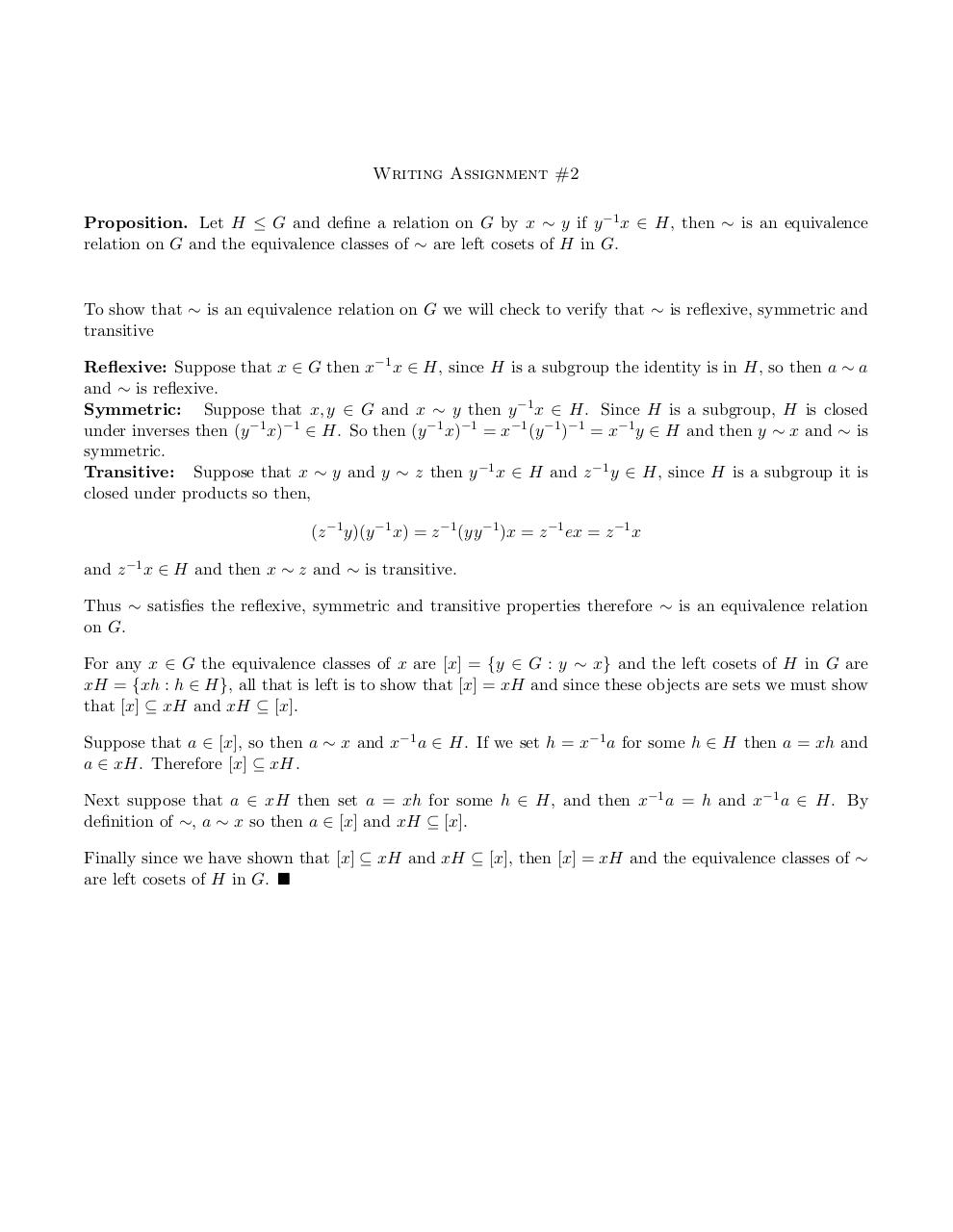# WR .pdf

### File information

Original filename: WR.pdf

This PDF 1.5 document has been generated by TeX / pdfTeX-1.40.14, and has been sent on pdf-archive.com on 24/04/2014 at 14:29, from IP address 198.39.x.x. The current document download page has been viewed 780 times.
File size: 81 KB (1 page).
Privacy: public file

## Download original PDF file

WR.pdf (PDF, 81 KB)

### Document preview

Writing Assignment #2
Proposition. Let H ≤ G and define a relation on G by x ∼ y if y −1 x ∈ H, then ∼ is an equivalence
relation on G and the equivalence classes of ∼ are left cosets of H in G.

To show that ∼ is an equivalence relation on G we will check to verify that ∼ is reflexive, symmetric and
transitive
Reflexive: Suppose that x ∈ G then x−1 x ∈ H, since H is a subgroup the identity is in H, so then a ∼ a
and ∼ is reflexive.
Symmetric: Suppose that x, y ∈ G and x ∼ y then y −1 x ∈ H. Since H is a subgroup, H is closed
under inverses then (y −1 x)−1 ∈ H. So then (y −1 x)−1 = x−1 (y −1 )−1 = x−1 y ∈ H and then y ∼ x and ∼ is
symmetric.
Transitive: Suppose that x ∼ y and y ∼ z then y −1 x ∈ H and z −1 y ∈ H, since H is a subgroup it is
closed under products so then,
(z −1 y)(y −1 x) = z −1 (yy −1 )x = z −1 ex = z −1 x
and z −1 x ∈ H and then x ∼ z and ∼ is transitive.
Thus ∼ satisfies the reflexive, symmetric and transitive properties therefore ∼ is an equivalence relation
on G.
For any x ∈ G the equivalence classes of x are [x] = {y ∈ G : y ∼ x} and the left cosets of H in G are
xH = {xh : h ∈ H}, all that is left is to show that [x] = xH and since these objects are sets we must show
that [x] ⊆ xH and xH ⊆ [x].
Suppose that a ∈ [x], so then a ∼ x and x−1 a ∈ H. If we set h = x−1 a for some h ∈ H then a = xh and
a ∈ xH. Therefore [x] ⊆ xH.
Next suppose that a ∈ xH then set a = xh for some h ∈ H, and then x−1 a = h and x−1 a ∈ H. By
definition of ∼, a ∼ x so then a ∈ [x] and xH ⊆ [x].
Finally since we have shown that [x] ⊆ xH and xH ⊆ [x], then [x] = xH and the equivalence classes of ∼
are left cosets of H in G.### Link to this page

#### Permanent link

Use the permanent link to the download page to share your document on Facebook, Twitter, LinkedIn, or directly with a contact by e-Mail, Messenger, Whatsapp, Line..

#### Short link

Use the short link to share your document on Twitter or by text message (SMS)

#### HTML Code

Copy the following HTML code to share your document on a Website or Blog

#### QR Code### Related keywords